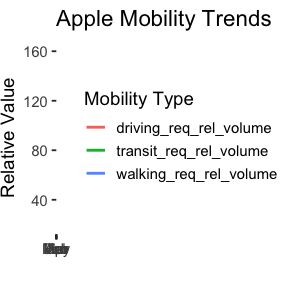# What are mobility trends like in the US?

Let’s load the data, and filter down to the United States of America.

library(covid19mobility)
library(dplyr)

us_mobile <- refresh_covid19mobility_apple_country() %>%
filter(location_code == "US")

Now, let’s plot it!

library(ggplot2)

ggplot(us_mobile,
aes(x = date, y = value, color = data_type)) +
geom_line(size = 1) +
theme_bw(base_size=14) +
labs(color = "Mobility Type",
y = "Relative Value",
x = "") +
ggtitle("Apple Mobility Trends")